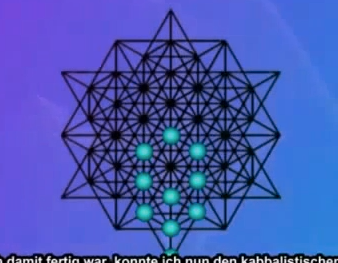top of page
Search

# Star tetrahedron tree of life

Enneagram=(star tetrahedron)=(tree of life)

(Star tetrahedron)=((3D) seed of life)

Enneagram=(fingerprint of god)(Fingerprint of god)=torus(equilateral triangle(energy)):Tetractys(tetragrammaton+10dimensions+(triangle numbers)+(tree of life))The 64 tetrahedron grid is made out of 8 tree of lifes(8 star tetrahedrons(8 tetragrammatons(tetractys)))All the superstrings that make up the infinite multyvers added up(all the superstrings harmonics added up) is -1/12 and all harmonics are encoded in a equilateral triangle(Equilateral  triangles are energy and waves(harmonics) and strings are 64 tetrahedron grids made out of energy in the form of a wave(harmonic)):

Star tetrahedrons:Atoms are energy in the form of a wave

(Xen particles)=nanites=strings

E8=(64 tetrahedron grid(2 tetragrammaton))